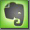Resistor Color Code

See this perfect online color code calculator by www.dannyg.com!

 Color A B C D E F silver 0.01 + - 10% gold 0.1 + - 5% black 0 0 0 1 brown 1 1 1 10 + - 1% 100ppm red 2 2 2 100 + - 2% 50ppm orange 3 3 3 1k 15ppm yellow 4 4 4 10k 25ppm green 5 5 5 100k + - 0.5% blue 6 6 6 1M + - 0.25% violet 7 7 7 10M + - 0.1% grey 8 8 8 + - 0.05% white 9 9 9

 4-band system 5-band system 6-band system A: first figure A: first figure A: first figure B: second figure B: second figure B: second figure D: multiplier C: third figure C: third figure E: tolerance D: multiplier D: multiplier E: tolerance E: tolerance F: temp. coeff.

Temperature Coefficient

The electrical resistance of a conductor is dependent upon collisional processes within the material. With increasing temperature the resistance will normally increase too.

The temperature coefficient of Copper is +0.393 percent per degree C (near room temperature): If the temperature increases 1 degree C the resistance will increase 0.393 percent.

0.3 percent per degree C equals '3300ppm per °C'.

The temperature coefficient of manufactured ohmic resistors ranges between 10ppm to 200ppm (regular resistors) and 7000ppm (temperature sensitive resistors).

Part Numbers Printed

Some resistors have the value and tolerance printed right on the side of the resistor itself. The alpha-numeric code means the following:

Example: RN60D1001F

RN: represents the type of resistor.
RN: high stability, fixed film resistor, RCR: carbon resistor, RW: fixed wire wound resistor, etc.

60: represents the power rating of the resistor (wattage).
60: the power rating is 1/8 watt, 10: 1/4 watt, 25: 1 watt, etc.

D: temperature coefficient (stated in PPM/°C).
D: 200 PPM/°C, etc.

1001: the ohmic value of the resistor.
The first three digits represent the ohmic value (here 100) and the last digit represents the number of zeros (here 1). For this example the value is: 100 Ohm x 1 Zero = 100 Ohm. The Number 8203 is: 820 Ohm x 3 Zeros ( = x 1000) = 820,000 Ohm = 820 kOhm. The R sign represents fractional values: The number 82R7 stands for 82.7 Ohm.

F: the tolerance of the resistor.
F: 1%, G: 2%, J: 5%, K: 10%, M: 20%.

The reliability and temperature coefficient bands are often not included. Both values together will not labelled on one resistor. Reliability indicates the failure rate per 100 hours.